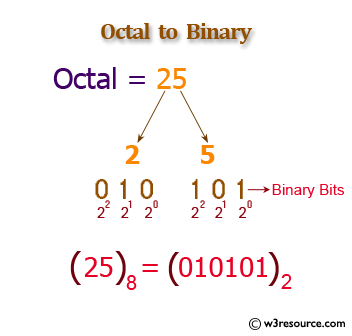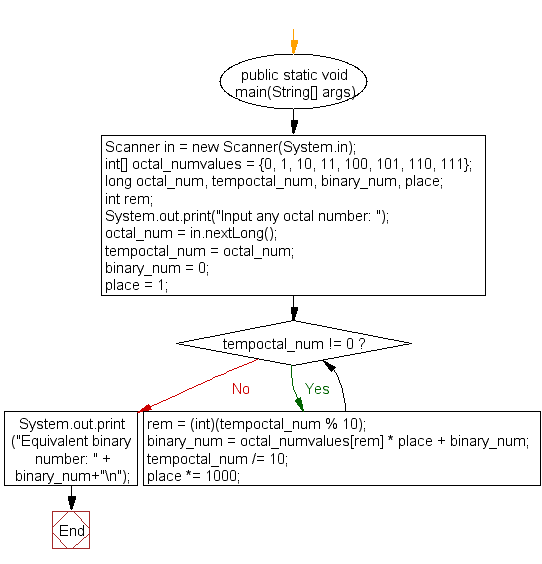﻿ Java exercises: Convert a octal number to a binary number - w3resource# Java Exercises: Convert a octal number to a binary number

## Java Basic: Exercise-26 with Solution

Write a Java program to convert a octal number to a binary number.

Octal number: The octal numeral system is the base-8 number system, and uses the digits 0 to 7.

Binary number: A binary number is a number expressed in the base-2 numeral system or binary numeral system. This system uses only two symbols: typically 1 (one) and 0 (zero).

Test Data:
Input any octal number: 7

Pictorial Presentation: Octal to Binary numberSample Solution:

Java Code:

``````import java.util.Scanner;

public class Exercise26 {
public static void main(String[] args)
{
Scanner in = new Scanner(System.in);
int[] octal_numvalues = {0, 1, 10, 11, 100, 101, 110, 111};
long octal_num, tempoctal_num, binary_num, place;
int rem;
System.out.print("Input any octal number: ");
octal_num = in.nextLong();
tempoctal_num = octal_num;
binary_num = 0;
place = 1;
while (tempoctal_num != 0)
{
rem = (int)(tempoctal_num % 10);
binary_num = octal_numvalues[rem] * place + binary_num;
tempoctal_num /= 10;
place *= 1000;
}
System.out.print("Equivalent binary number: " + binary_num+"\n");
}
}
```
```

Sample Output:

```Input any octal number: 7
Equivalent binary number: 111
```

Flowchart:Java Code Editor:

What is the difficulty level of this exercise?

Test your Programming skills with w3resource's quiz.

﻿

## Java: Tips of the Day

getEnumMap

Converts to enum to Map where key is the name and value is Enum itself.

```public static <E extends Enum<E>> Map<String, E> getEnumMap(final Class<E> enumClass) {
return Arrays.stream(enumClass.getEnumConstants())
.collect(Collectors.toMap(Enum::name, Function.identity()));
}
```

Ref: https://bit.ly/3xXcFZt